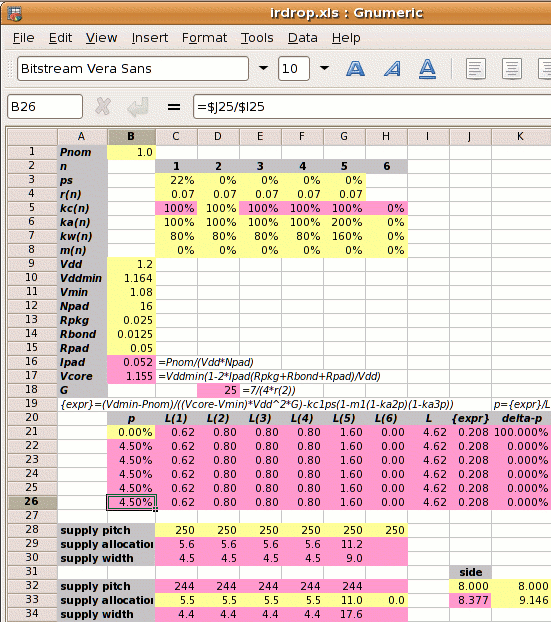Step 1: Calculate Ipad and Vcore:

1⁄(1.2×16) = 0.052A
Vcore =
1.164×(1−2×0.052×(0.025+0.0125+0.05)⁄1.2
1.155V

Step 2: Calculate the reference power supply conductance G:

G =
 7 4×r2

7 ⁄ (4 × 0.07) =  25 mhos

Step 3 is to set out the values of kan, kwn, kcn and mn for each metal layer, and use these to calculate the value of L.

metal layer 1 2 3 4 5 6
kan 100% 100% 100% 100% 100%   0%¹
power metal allocated coefficient
kwn  80%  80%  80%  80%  80%   0%¹
power metal used coefficient
kcn 100% 100% 100% 100% 100%   0%¹
conductivity coefficient
mn²   0%   0%   0%   0%   0%   0%
core area blocked
¹no metal-6 layer; ²no fixed blocks

Normally we can't calculate L directly because its value depends on p which we don't know. But in this case with m1‑6=0 we can

 L = kw1kc1(1-ps)(1-m1(1-ka2p)(1-ka3p))+ kw2kc2(1-m2(1-ka2p)(1-ka3p))+ kw3kc3(1-m3(1-ka2p)(1-ka3p))+ kw4kc4(1-m4(1-ka2p)(1-ka3p))+ kw5kc5(1-m5(1-ka2p)(1-ka3p)) = ( 0.62 + 0.8 + 0.8 + 0.8 + 1.6 ) = 4.62

Step 4: Calculate the power strap allocation percentage p:

p =
 { Vddmin×Pnom −kc1×ps } × 1 (Vcore−Vmin)×Vdd2×G L
 { 1.14×1 −1×0.22 } × 1 (1.125−1.08)×1.22×25 4.62
(0.430−0.222)×0.216 = 4.50%

As shown on the right, a spreadsheet can be used to iterate to the answer.

Step 5: Calculate the new core size. If the initial core size estimate without power straps is x, then with power straps the core size becomes x

 x′ = x = x = x = x+4.71% √(((1-ka2p)(1-ka3p)) √0.9552 0.955

The value 4.71% is called the IR Drop Adder.

The width of the power straps is set by the pitch, strap allocation or width chosen by the user and the value of p just calculated. An example is shown in the table below, where we set the supply strap allocation to 5.5µm and compare it to the old solution.

p allocation
met‑1‑4
allocation
met‑5
pitch   core side
old 12.53% 5.5µm 5.5µm  88µm 9.146mm
new  4.50% 5.5µm 11µm 244µm 8.377mm

Design Attribute Value
Pnom core power consumption 1W
ps fraction of metal-1 in the standard cells used for power supplies 22% (for vsclib)
rn resistivity of metal layer n in ohms per square 0.07Ω per sq.
kan
 user defined   ratio of metal layer n allocated to power metal-2 allocated to power
100%
kwn
 user defined   ratio of metal layer n used for power metal-2 allocated to power
80%
mn percentage of metal layer n blocked to power straps 0%
Vdd the nominal supply voltage 1.2V
Vddmin the minimum supply voltage, 3% less than nominal 1.164V
Vmin the desired voltage at the centre of the die, 10% less than the nominal 1.08V
Rpkg the resistance of the package leadframe 25mΩ
Rbond the resistance of the bond wire 12.5mΩ¹
¹double bond halves bond wire resistance; ²two supply pads halves pad resistance

kcn =
 r2 rnThe new solution has allowed the power strap pitch to go up from one every 88µm to one every 244µm. The core side is 769µm less and the core area is 16% less than the first solution, due to double bonding of supply pads, tighter Vddmin spec and wider metal-5 straps.